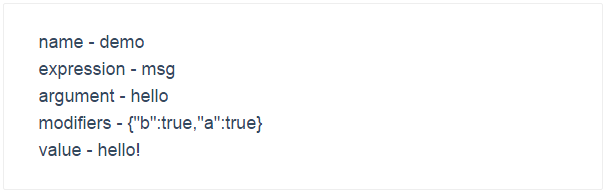# 自定义指令

## 基础

### 钩子函数

• bind：只调用一次，在指令第一次绑定到元素上时调用。

• update： 在 `bind` 之后立即以初始值为参数第一次调用，之后每当绑定值变化时调用，参数为新值与旧值。

• unbind：只调用一次，在指令从元素上解绑时调用。

``````Vue.directive('my-directive', {
bind: function () {
// 准备工作
// 例如，添加事件处理器或只需要运行一次的高耗任务
},
update: function (newValue, oldValue) {
// 值更新时的工作
// 也会以初始值为参数调用一次
},
unbind: function () {
// 清理工作
// 例如，删除 bind() 添加的事件监听器
}
})
``````

``````<div v-my-directive="someValue"&gt;</div&gt;
``````

``````Vue.directive('my-directive', function (value) {
// 这个函数用作 update()
})
``````

### 指令实例属性

• el: 指令绑定的元素。
• vm: 拥有该指令的上下文 ViewModel。
• expression: 指令的表达式，不包括参数和过滤器。
• arg: 指令的参数。
• name: 指令的名字，不包含前缀。
• modifiers: 一个对象，包含指令的修饰符。
• descriptor: 一个对象，包含指令的解析结果。

``````<div id="demo" v-demo:hello.a.b="msg"&gt;</div&gt;
``````
``````Vue.directive('demo', {
bind: function () {
console.log('demo bound!')
},
update: function (value) {
this.el.innerHTML =
'name - '       + this.name + '<br&gt;' +
'expression - ' + this.expression + '<br&gt;' +
'argument - '   + this.arg + '<br&gt;' +
'modifiers - '  + JSON.stringify(this.modifiers) + '<br&gt;' +
'value - '      + value
}
})
var demo = new Vue({
el: '#demo',
data: {
msg: 'hello!'
}
})
``````### 对象字面量

``````<div v-demo="{ color: 'white', text: 'hello!' }"&gt;</div&gt;
``````
``````Vue.directive('demo', function (value) {
console.log(value.color) // "white"
console.log(value.text) // "hello!"
})
``````

### 字面修饰符

``````<div v-demo.literal="foo bar baz"&gt;
``````
``````Vue.directive('demo', function (value) {
console.log(value) // "foo bar baz"
})
``````

### 元素指令

``````Vue.elementDirective('my-directive', {
// API 同普通指令
bind: function () {
// 操作 this.el...
}
})
``````

``````<div v-my-directive&gt;</div&gt;
``````

``````<my-directive&gt;</my-directive&gt;
``````

## 高级选项

### params

``````<div v-example a="hi"&gt;</div&gt;
``````
``````Vue.directive('example', {
params: ['a'],
bind: function () {
console.log(this.params.a) // -&gt; "hi"
}
})
``````

``````<div v-example v-bind:a="someValue"&gt;</div&gt;
``````
``````Vue.directive('example', {
params: ['a'],
paramWatchers: {
a: function (val, oldVal) {
console.log('a changed!')
}
}
})
``````

### deep

``````<div v-my-directive="obj"&gt;</div&gt;
``````
``````Vue.directive('my-directive', {
deep: true,
update: function (obj) {
// 在 `obj` 的嵌套属性变化时调用
}
})
``````

### twoWay

``````Vue.directive('example', {
twoWay: true,
bind: function () {
this.handler = function () {
// 将数据写回 vm
// 如果指令这样绑定 v-example="a.b.c"
// 它将用给定值设置 `vm.a.b.c`
this.set(this.el.value)
}.bind(this)
},
unbind: function () {
this.el.removeEventListener('input', this.handler)
}
})
``````

### acceptStatement

``````<div v-my-directive="a++"&gt;</div&gt;
``````
``````Vue.directive('my-directive', {
acceptStatement: true,
update: function (fn) {
// 传入值是一个函数
// 在调用它时将在所属实例作用域内计算 "a++" 语句
}
})
``````